# AIIMS Full Mock Test 19

## 200 Questions MCQ Test AIIMS Mock Tests & Previous Year Papers | AIIMS Full Mock Test 19

Description
This mock test of AIIMS Full Mock Test 19 for NEET helps you for every NEET entrance exam. This contains 200 Multiple Choice Questions for NEET AIIMS Full Mock Test 19 (mcq) to study with solutions a complete question bank. The solved questions answers in this AIIMS Full Mock Test 19 quiz give you a good mix of easy questions and tough questions. NEET students definitely take this AIIMS Full Mock Test 19 exercise for a better result in the exam. You can find other AIIMS Full Mock Test 19 extra questions, long questions & short questions for NEET on EduRev as well by searching above.
QUESTION: 1

Solution:
QUESTION: 2

Solution:
QUESTION: 3

### If the rms current in a 50 Hz ac circuit is 5A, the value of the current 1/300 seconds after its value becomes zero is :

Solution:
QUESTION: 4

When a voltage measuring device is connected to AC mains, the meter shown the steady input voltage of 220V. This means

Solution:
QUESTION: 5

A rectangular coil of 300 turns has an average area of 25cm × 10cm. The coil rotates with a speed of 50 cps in a uniformmagnetic field of strength 4×10-2 T about an axis perpendicular of the field. The peak value of the induced e.m.f. is (in volt)

Solution:
QUESTION: 6

A solenoid has 2000 turns wound over a length of 0.30 meter. The area of its cross-section is 1.2×10-3 m2, Around its central section, a coil of 300 turns is wound. If an initial current of 2A in the solenoid is reversed in 0.25 sec, then the e.m.f. induced in the coil is

Solution:
QUESTION: 7

An ideal coil of 10 henry is joined in series with a resistance of 5 ohm and a battery of 5 volt. 2 sec after joining, the current flowing in ampere in the circuit will be

Solution:
QUESTION: 8

The adjoining figure shows two bulbs B1 and B2 resistor R and and inductor L.When the switch S is turned off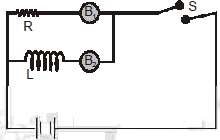Solution:
QUESTION: 9

Two conducting circular loops of radii R1 and R2 are placed in the same plane with their centres coinciding. If R1 >> R2, the mutual inductance M between them will be directly proportional to

Solution:
QUESTION: 10

A thin semicircular conducting ring of radius R is falling with its plane vertical in a horizontalmagnetic induction B. At the position MNQ, the speed of the ring is V and the potential difference developed across the ring is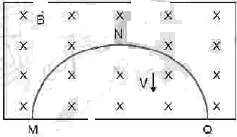Solution:
QUESTION: 11

A uniform but time-varying magnetic field B(t) exists circular region of radius a and is directed into the plane of the paper, as shown. The magnitude of the induced electric field at point P at a distance r from the center of the circle region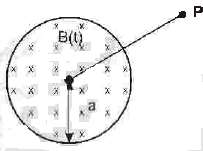Solution:
QUESTION: 12

When a certain circuit consisting of a constant e.m.f. E an inductance L and a resistance R is closed, the current in, it increases with time according to curve 1. After one parameter (E,L or R) is changed, the current in the circuit increases with time according to curve 2. Which parameter was changed and in what direction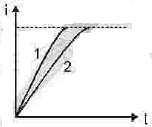Solution:
QUESTION: 13

When the number of turns and the length of the solenoid are doubled keeping the area of cross section same, the inductance

Solution:
QUESTION: 14

In the ideal oscillating circuit, the capacitance of the capacitor is 25 μF and has initial charge of 30 μC. The inductance of coil is 0.04 H. The maximum magni de of current in the circuit, after closing the switch K is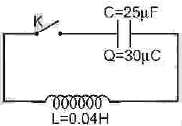Solution:
QUESTION: 15

An alternating current of frequency ‘f’ is flowing in a circuit containing a resistance R and a choke L in series. The impedance of this circuit is

Solution:
QUESTION: 16

A resonant ac circuit contains a capacitor of capacitance 10-6 F and an inductor of 10-4 H. The frequency of electrical oscillations will be

Solution:
QUESTION: 17

A resistance of 300 Ω and an inductance of 1/π  henry are connected in series to a ac voltage of 20 volts and 200 Hz frequency. The phase angle between the voltage and current is

Solution:
QUESTION: 18

An alternating voltage E = 200√2 sin (100 t) is connected to a 1 microfarad capacitor through an ac ammeter. The reading of the ammeter shall be

Solution:
QUESTION: 19

A 220 V, 50 Hz ac source is connected to an inductance of 0.2 H and a resistance of 20 ohm in series. What is the current in the circuit

Solution:
QUESTION: 20

In the circuit shown below, the ac source has voltage V = 20 cos (ωt) volts with ω = 2000 rad/sec. The amplitude of the current will be earest to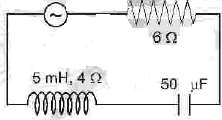Solution:
QUESTION: 21

In a circuit L,C and R are connected in series with an alternating voltage source of frequency f . The current leads the voltage by 45°. The value of C is

Solution:
QUESTION: 22

Which one of the following curves represents the variation of impedance (Z) with frequency f in series LCR circuit

Solution:
QUESTION: 23

A constant voltage at different frequencies is applied across a capacitance C as shown in the figure. Which of the following graphs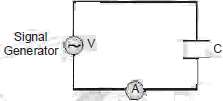Correctly depicts the variation of current with frequency?

Solution:
QUESTION: 24

The figure shows variation of R, XL and XC with frequency f in a series L, C, R circuit. Then for what frequency point, the circuit is inductive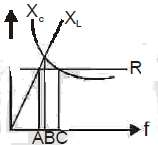Solution:
QUESTION: 25

In which of the following circuit is the current maximum just after the switch S is closed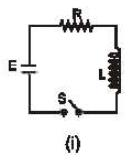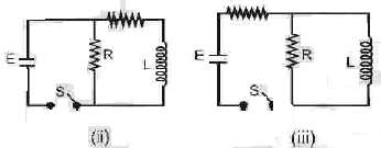Solution:
QUESTION: 26

A small coil is introduced between the poles of an electromagnet so that its axis coincides with the magnetic field direction. The number of turns is n and the cross sectional area of the coil is A.When the coil turns through 1800 about its diameter, the charge flowing through the coil is Q. The total resistance of the circuit is R. What is the magnitude of the magnetic induction

Solution:
QUESTION: 27

Two circular coils A and B are facing each other as shown in figure. The current i through A can be altered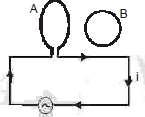Solution:
QUESTION: 28

A conducting loop having a capacitor is moving outward from the magnetic field then which plate of the capacitor will be positive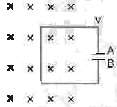Solution:
QUESTION: 29

A straight wire of length L is bent into a semicircle. It is moved in a uniform magnetic field with speed v with diameter perpendicular to the field. The induced emf between the ends of the wire is

Solution:
QUESTION: 30

If in a coil rate of change of area is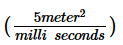and current become 1 amp form 2 amp in 2 x 10-3sec. If magnetic field is 1 Tesla then self inductance of the coil is

Solution:
QUESTION: 31

A pair of parallel conducting rails lie at right angle to a uniform magnetic field of 2.0 T as shown in the fig. Two resistors 10 Ω and 5 Ω are to slide without friction along the rail. The distance between the conducting rails is 0.1 m. Then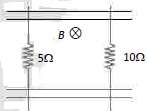Solution:
QUESTION: 32

A hundred turns of insulated copper wire are wrapped a ound an iron cylinder of area 1×10-3 m2 and are connected to a resistor. The total resistance in the circuit is 10 ohms. If the longitudinal magnetic induction in the iron change from 1 weber m-2, in one direction to 1 Weber m-2 in the opposite direction, how much charge flows through the circuit

Solution:
QUESTION: 33

In an LCR circuit R = 100 ohm. When capacitance C is removed, the current lags behind the voltage by π = 3. When inductance L is removed, the current leads t e voltage by π/3. The impedance of the circuit is

Solution:
QUESTION: 34

A group of electric lamps having a total power rating of 1000 watt is supplied by an ac voltage. E = 200sin (310t + 600). Then the r.m.s. value of the circuit current is

Solution:
QUESTION: 35

Following figure shows an ac generator connected to a "block box" through a pair of terminals. The box contains possible R, L, C or their combination, whose elements and arrangements are not known to us. Measurements outside the box reveals that e = 75 sin (sin ωt) volt, i = 1.5 sin ( ωt + 450) amp then, the wrong statement is

Solution:
QUESTION: 36

An alternating current of frequency 200 rad/sec and per k value 1A as shown in the figure, is applied to the primary of a transformer. If the coefficient of mutual induction between the primary and the secondary is 1.5 H, the voltage induced in the secondary will be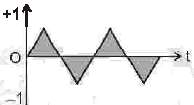Solution:
QUESTION: 37

A resistor R, an inductor L and a capacitor C are conn cted in series to an oscillator of frequency n. if the resonant frequency is nr, then the current lags behind voltage, when

Solution:
QUESTION: 38

The figure shows different graphs between stopping potential (V0) and frequency (V ) for photosensitive surface of cesium, potassium, sodium and lithium. The plots are parallel. Correct ranking of the targets according to their work function greatest first will be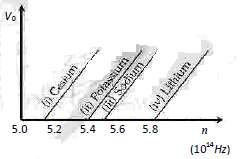Solution:
QUESTION: 39

Five elements A, B, C, D and E have work functions 1.2 eV, 2.4 eV, 3.6 eV, 4.8 eV and 6 eV respectively. If light of wave length 4000 Å is allowed to fall on these elements, then photo electrons are emitted by

Solution:
QUESTION: 40

If light of wavelength λ1 is allowed to fall on a metal, then kinetic energy of photoelectrons emitted is E1 . If wavelength of light changes to λ2 then kinetic energy of electrons changes to E2 . Then work function of the metal is

Solution:
QUESTION: 41

The following questions consist of two statements one labelled ASSERTION (A) and the another labelled REASON (R). Select the correct answers to these questions from the codes given below :

Assertion: Work function of aluminium is 4.2 eV. Emiss on of electrons will not be possible if two photons each of energy 2.5 eV collide with free electrons of aluminium.
Reason : For photoelectric emission the energy of each photon should be greater than or equal to the work function of aluminium

Solution:
QUESTION: 42

The following questions consist of two statements one labelled ASSERTION (A) and the another labelled REASON (R). Select the correct answers to these questions from the codes given below :

Assertion: Kinetic energy of photo electrons emitted by a photosensitive surface depends upon the intensity of incident photon.
Reason : The ejection of electrons from metallic surface is possible with frequency of incident photon below the threshold frequency.

Solution:
QUESTION: 43

The following questions consist of two statements one labelled ASSERTION (A) and the another labelled REASON (R). Select the correct answers to these questions from the codes given below :

Assertion : Photosensitivity of a metal is high if its work function is small.
Reason : Work function = hf0 where f0 is the threshold frequency

Solution:
QUESTION: 44

The following questions consist of two statements one labelled ASSERTION (A) and the another labelled REASON (R). Select the correct answers to these questions from the codes given below :

Assertion : Average power consumed in an ac circuit is equal to average power consumed by resistors in the circuit.
Reason : Average power consumed by capacitor and inductor is zero

Solution:
QUESTION: 45

The following questions consist of two statements one labelled ASSERTION (A) and the another labelled REASON (R). Select the correct answers to these questions from the codes given below :

Assertion : The electrostatic energy stored in capacitor plus magnetic energy stored in inductor will always be zero in a series LCR circuit driven by ac voltage source under condition of resonance.
Reason : The complete voltage of ac source appears eqully across the all the elements in a series LCR circuit driven by ac voltage source under condition of resonance

Solution:
QUESTION: 46

The following questions consist of two statements one  labelled ASSERTION (A) and the another labelled REASON (R). Select the correct answers to these questions from the codes given below :

Assertion : Two coaxial conducting rings of different radii are placed concentric in space. The mutual inductance of both the rings is maximum if the rings are also coplanar.
Reason : For two coaxial conducting rings of different radii, the magnitude of magnetic flux in one ring due to current in other ring is maximum when both rings are coplanar.

Solution:
QUESTION: 47

The following questions consist of two statements one labelled ASSERTION (A) and the another labelled REASON (R). Select the correct answers to these questions from the codes given below :

Assertion : Only a change in magnetic flux will maintain an induced current the coil.
Reason : The presence of large magnetic flux through a coil maintains a current in the coil if the circuit is continuous.

Solution:
QUESTION: 48

The following questions consist of two statements one labelled ASSERTION (A) and the another labelled REASON (R). Select the correct answers to these questions from the codes given below :

Assertion : Self-inductance is called the inertia of electricity.
Reason : Self-inductance is the phenomenon, according which an opposing induced e.m.f. is produced in a coil as a result of change in current or magnetic flux linked in the coil.

Solution:
QUESTION: 49

The following questions consist of two statements one labelled ASSERTION (A) and the another labelled REASON (R). Select the correct answers to these questions from the codes given below :

Assertion :When two coils arewound on each other, themutual induction between the coils is maximum.
Reason: Mutual induction does not depend on the orientation of the coils.

Solution:
QUESTION: 50

The following questions consist of two statements one labelled ASSERTION (A) and the another labelled REASON (R). Select the correct answers to these questions from the codes given below :

Assertion : Acceleration of a magnet falling through a long solenoid decreases.
Reason: The induced current produced in a circuit always flow in such direction that it opposes the change or the cause the produced it.

Solution:
QUESTION: 51

The following questions consist of two statements one labelled ASSERTION (A) and the another labelled REASON (R). Select the correct answers to these questions from the codes given below :

Assertion : A bar magnet is dropped into a long vertical copper tube. Even taking air resistance as negligible, the magnet attains a constant terminal velocity. If the tube is heated, the terminal velocity gets increased.
Reason : The terminal velocity depends on eddy current produced in bar magnet

Solution:
QUESTION: 52

The following questions consist of two statements one labelled ASSERTION (A) and the another labelled REASON (R). Select the correct answers to these questions from the codes given below :

Assertion : Ca pacitor serves as a block for dc and offers an easy path to ac.
Reason : Capacitive reactance is inversely proportional to frequency.

Solution:
QUESTION: 53

The following questions consist of two statements one labelled ASSERTION (A) and the another labelled REASON (R). Select the correct answers to these questions from the codes given below :

Assertion : When capacitive reactance is smaller than the inductive reactance in LCR current, e.m.f. leads the current .
Reason : The phase angle is the angle between the alternating e.m.f. and alternating current of the circuit

Solution:
QUESTION: 54

The following questions consist of two statements one labelled ASSERTION (A) and the another labelled REASON (R). Select the correct answers to these questions from the codes given below :

Assertion : Chock coil is preferred over a resistor to adjust current in an ac circuit.
Reason : Power factor for inductance is zero.

Solution:
QUESTION: 55

The following questions consist of two statements one labelled ASSERTION (A) and the another labelled REASON (R). Select the correct answers to these questions from the codes given below :

Assertion : If the frequency of alternating current in an ac circuit consisting of an inductance coil is increased then current gets decreased.
Reason : The current is inversely proportional to frequency of alternating current.

Solution:
QUESTION: 56

The following questions consist of two statements one labelled ASSERTION (A) and the another labelled REASON (R). Select the correct answers to these questions from the codes given below :

Assertion : On introducing soft iron core in the solenoid, the inductance increases.
Reason : A bulb connected in series with a solenoid is connected to ac source. If a soft iron core is introduced in the solenoid, the bulb will glow brighter.

Solution:
QUESTION: 57

The following questions consist of two statements one labelled ASSERTION (A) and the another labelled REASON (R). Select the correct answers to these questions from the codes given below :

Assertion : The complete voltage of ac source appears across the resistor in a series LCR circuit driven by ac voltage source under condition of resonance.
Reason : The electrostatic energy stored in capacitor plus magnetic energy stored in inductor will always be zero in a series LCR circuit driven by ac voltage source under condition of resonance.

Solution:
QUESTION: 58

The following questions consist of two statements one labelled ASSERTION (A) and the another labelled REASON (R). Select the correct answers to these questions from the codes given below :

Assertion : Average power consumed in an ac circuit is equal to average power consumed by resistors in the circuit.
Reason : Average power consumed by capacitor and inductor is zero

Solution:
QUESTION: 59

The following questions consist of two statements one labelled ASSERTION (A) and the another labelled REASON (R). Select the correct answers to these questions from the codes given below :

Assertion : Average power consumption by a capacitor in one cycle for a sinusoidally varying ac current is zero.
Reason : The power consumed by a capacitor in a sinusoidally varying ac circuit is zero for every small time interval.

Solution:
QUESTION: 60

The following questions consist of two statements one labelled ASSERTION (A) and the another labelled REASON (R). Select the correct answers to these questions from the codes given below :

Assertion : An inductor is connected to an ac source.When the magnitude of current decreases in the circuit, energy is absorbed by the ac source.
Reason : When current through an inductor decreases, the energy stored in inductor decreases

Solution:
QUESTION: 61

The solubility of AgBr in 0.4 M NaBr is :  (Ksp, AgBr = 4 × 10-13)

Solution:
QUESTION: 62

pH of 0.1 M (NH4)2C2O4 is 6.8. If 10 ml of water is added to it, pH becomes

Solution:
QUESTION: 63

What is the difference in pH for 1/3 & 2/3 stages of neutralization of 0.1 M CH3COOH with 0.1 M NaOH

Solution:
QUESTION: 64

An acid indicator is represented by HIn. (Ka = 10-5). The range of change of colour for the indicator is

Solution:
QUESTION: 65

Which has maximum solubility AB, AB2, AB3 and AB4 if KSP for all the salts are 10-10 :

Solution:
QUESTION: 66

Three bases XOH, YOH & ZOH has pKb values 2, 3 & 4 respectively the strongest conjugate acid is :

Solution:
QUESTION: 67

The ambidentate as well as monodentate ligand is :

Solution:
QUESTION: 68

The oxidation state of Fe in brown ring complex [Fe(H2O)5NO]SO4 is :

Solution:
QUESTION: 69

The primary and secondary valencies of chromium according to the Werner theory in the complex ion, dichloridodioxalatochromium (III) are respectively :

Solution:
QUESTION: 70

The EAN of platinum in potassium hexachloridoplatinate(IV) is: [Pt (z=78)]

Solution:
QUESTION: 71

Which of the following is non–conducting in its aquous solution ?

Solution:
QUESTION: 72

In which of the following configurations will there be the possibility of both paramagnetism and diamagnetism, depending on the nature of the ligands?

Solution:
QUESTION: 73

A complex cation is formed by Pt (in some oxidation state) with ligands (in proper number so that coordination number of Pt becomes six). Which of the following can be its correct IUPAC name :

Solution:
QUESTION: 74

For which of the following complexes, the crystal field stabilising energy will be maximum

Solution:
QUESTION: 75

Amongst the following the most stable complex is :

Solution:
QUESTION: 76

Which of the following complex show ionization, linkage and geometrical isomerism ?
(I) [Co(NH3)5(NO2)] Br ;
(II) [Co(NH3)5(H2O)] (NO2)2
(III) [Pt(NH3)4] [Pt(SCN)4] ;
(IV) [Cr(NH3)4 (NO2)2](NO3)

Solution:
QUESTION: 77

For an ionic solid of general formula AB and coordination number 8 for both A and B, the value of radius ratio will be :

Solution:
QUESTION: 78

The lattice of CaF2 is called fluorite structure. SrCl2 has fluorite structure. Which of the following statements is true for SrCl2 ?

Solution:
QUESTION: 79

Schottky defect occurs mainly in electrovalent compounds where

Solution:
QUESTION: 80

The cubic unit cell of Al(molar mass 27 g mol-1) has an edge length of 405 pm. Its density is 2.7 g cm-3. The cubic unit cell is :

Solution:
QUESTION: 81

A particular solid is very hard and has a very high melting point. In solid state, it is a non conductor and its melt is a conductor of electricity. Classify the solid.

Solution:
QUESTION: 82

In AgBr, there can occur

Solution:
QUESTION: 83

In an antifluorite structure, cations occupy :

Solution:
QUESTION: 84

For an octahedral complex of Co(III) , Δ0 = 25000 Cm-1 and electron pair energy (P) = 15000Cm-1. The total numerical value of CFSE in KJmol-1 will be nearly ?

Solution:
QUESTION: 85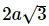The interionic distance for cesium chloride crystal will be :

Solution:
QUESTION: 86

With the help of following data predict the colour of the complex ion [Ti(H2O)6]3+.CFSE = –83.2 KJ mole-1.The colour wheel with in nm is given as : The colour can be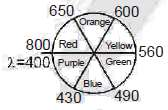Solution:
QUESTION: 87

In which of the following species C–O bond length is maximum?

Solution:
QUESTION: 88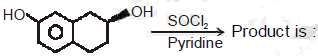Solution:
QUESTION: 89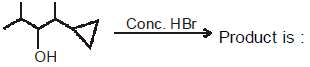Solution:
QUESTION: 90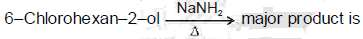Solution:
QUESTION: 91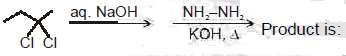Solution:
QUESTION: 92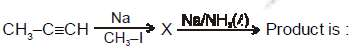Solution:
QUESTION: 93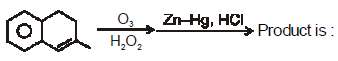Solution:
QUESTION: 94

In which reaction major product is correct ?

Solution:
QUESTION: 95

Rate of Hydrolysis (SN1) reaction for the given molecules is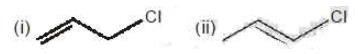(iii) Ph-CH2-Cl              (iv)   CH3 - CH2-CH2-Cl

Solution:
QUESTION: 96

Which of the following compounds will give racemic mixture on nucleophilic substitution by OH- ion?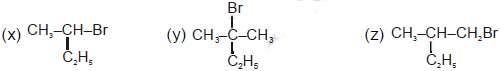Solution:
QUESTION: 97

Which of the following statement/s is/are correct about the mechanism of this reaction?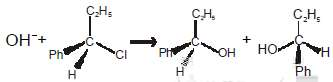Solution:
QUESTION: 98

The hydrolysis products P and Q can be distinguished by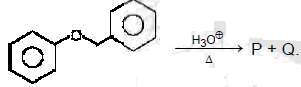Solution:
QUESTION: 99

The product Q of the following reaction is :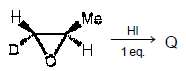Solution:
QUESTION: 100

For the given reaction, rate of substitution is studied for different R-groups. The rate is expected to be in this order (for different R-group).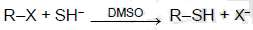Solution:
QUESTION: 101

The following questions consist of two statements one labelled ASSERTION (A) and the another labelled REASON (R). Select the correct answers to these questions from the codes given below :

Assertion : solubility of BaSO4 in 0.1 M Na2SO4 is 10-9 M hence its KSP is 10-10
Reason : for BaSO4 KSP = (s)2 in pure water

Solution:
QUESTION: 102

The following questions consist of two statements one labelled ASSERTION (A) and the another labelled REASON (R). Select the correct answers to these questions from the codes given below :

Assertion : Aqueous solution of CH3COONH4 is found to be neutral.
Reason : because this salt does not undergo hydrolysis

Solution:
QUESTION: 103

The following questions consist of two statements one labelled ASSERTION (A) and the another labelled REASON (R). Select the correct answers to these questions from the codes given below :

Assertion : The dissociation constants of polyprotic acid are in the oder K1 > K2 > K3 .
Reason : The [H+] furnished in first step of dissociation is high and exerts common ion effect to reduce the second dissociation so on.

Solution:
QUESTION: 104

The following questions consist of two statements one labelled ASSERTION (A) and the another labelled REASON (R). Select the correct answers to these questions from the codes given below :

Assertion : A mixture of the solutions of a weak acid and its conjugate base acts as a good buffer.
Reason : The ratio of the conjugates base to acid in the mixture does not change substantially when small amount of acids or alkalies are added to the buffer

Solution:
QUESTION: 105

The following questions consist of two statements one labelled ASSERTION (A) and the another labelled REASON (R). Select the correct answers to these questions from the codes given below :

Assertion : Acetylacetone complexes of the metal ions are more stable than the corresponding ethylene-diamine complexes.
Reason : Acetylacetone complexes of the metal ions contain a six membered conjugate chelate rings and the resonance in its chelate ion is an additional factor contributing to the stability

Solution:
QUESTION: 106

The following questions consist of two statements one labelled ASSERTION (A) and the another labelled REASON (R). Select the correct answers to these questions from the codes given below :

Assertion : Complexes, [Ti(H2O)6]Cl3 and [Ti(H2O)5Cl]Cl2 will be of same colour.
Reason : CFSE for both these will be same

Solution:
QUESTION: 107

The following questions consist of two statements one  labelled ASSERTION (A) and the another labelled REASON (R). Select the correct answers to these questions from the codes given below :

Assertion :All the complexes of Pt (+II ) andAu(+III ) (C.No = 4) are generally square planar geometry.
Reason : The crystal field splitting o is larger for second and third row transition elements , and for more highly charged species. This larger value of Δ0 energetically favours the pairing of electron for tetrahedal.

Solution:
QUESTION: 108

The following questions consist of two statements one labelled ASSERTION (A) and the another labelled REASON (R). Select the correct answers to these questions from the codes given below :

Assertion : Potassium ferrocyanide is diamagnetic, whereas potassiumferricyanide is paramagnetic.
Reason : Crystal field splitting in ferrocyanide ion is greater than that of ferricyanide ion.

Solution:
QUESTION: 109

The following questions consist of two statements one labelled ASSERTION (A) and the another labelled REASON (R). Select the correct answers to these questions from the codes given below :

Assertion : [Ni(CN)4]2- is a square planar complex.
Reason : [CN]- is a strong ligand.

Solution:
QUESTION: 110

The following questions consist of two statements one labelled ASSERTION (A) and the another labelled REASON (R). Select the correct answers to these questions from the codes given below :

Assertion : In HCP structure the contribution of hexagonal face corner per atom is 1/12.
Reason : It is shared by 12 different unit cell.

Solution:
QUESTION: 111

The following questions consist of two statements one labelled ASSERTION (A) and the another labelled REASON (R). Select the correct answers to these questions from the codes given below :

Assertion : ZnO becomes yellow when it is heated.
Reason : NaCl becomes yellow when heated in the presence of Na vapours due to anion vacancy.

Solution:
QUESTION: 112

The following questions consist of two statements one labelled ASSERTION (A) and the another labelled REASON (R). Select the correct answers to these questions from the codes given below :

Assertion : In NaCl crystal each Na+ ion is touching 6 Cl- ions but these Cl- ions do not touch each other.  Reason : The radius ratio rNa+ /rCl- is greater than 0.414, required for exact fitting.

Solution:
QUESTION: 113

The following questions consist of two statements one labelled ASSERTION (A) and the another labelled REASON (R). Select the correct answers to these questions from the codes given below :

Assertion : A solution of [Ni (H2O)6]2+ is green but a solution of [Ni(CN)4]2- is colourless .
Reason : Energy difference between d levels (i.e.Δ ) for H2O complex is in the visible region and that for the cyano complex is in the UV region.

Solution:
QUESTION: 114

The following questions consist of two statements one labelled ASSERTION (A) and the another labelled REASON (R). Select the correct answers to these questions from the codes given below :

Assertion: KCN and AgCN reacts with methyl chloride to give Ethane nitrile and methyl isocyanide respectively.
Reason : KCN has more covalent nature than AgCN

Solution:
QUESTION: 115

The following questions consist of two statements one labelled ASSERTION (A) and the another labelled REASON (R). Select the correct answers to these questions from the codes given below :

Assertion : Hydrolysis of (–)-2-bromooctane proceeds with inversion of configuration.
Reason : This reaction proceeds through the formation of a carbocation

Solution:
QUESTION: 116

The following questions consist of two statements one labelled ASSERTION (A) and the another labelled REASON (R). Select the correct answers to these questions from the codes given below :

Assertion : Among isomeric butyl alcohols, sec-butyl alcohol exhibits enantiomerism
Reason : Sec-butyl alcohol has a chiral carbon atom.

Solution:
QUESTION: 117

The following questions consist of two statements one labelled ASSERTION (A) and the another labelled REASON (R). Select the correct answers to these questions from the codes given below :

Assertion : Di-tert-butyl ether can be prepared by Williamson synthesis.
Reason : Tert-butyl bromide on treatment with sodium tert-butoxide preferentially undergoes substitution reaction

Solution:
QUESTION: 118

The following questions consist of two statements one labelled ASSERTION (A) and the another labelled REASON (R). Select the correct answers to these questions from the codes given below :

Assertion : Allyl bromide undergoes nucleophilic substitution much more readily than vinyl bromide.
Reason : C–Br bond in vinyl bromide has partial double bond character due to resonance.

Solution:
QUESTION: 119

The following questions consist of two statements one labelled ASSERTION (A) and the another labelled REASON (R). Select the correct answers to these questions from the codes given below :

Assertion: Reaction with aqueous NaOH of ethyl chloride and ethylidene chloride give different products.
Reason : Products obtained in the above reactions can be distinguished by Tollen's reagent.

Solution:
QUESTION: 120

The following questions consist of two statements one labelled ASSERTION (A) and the another labelled REASON (R). Select the correct answers to these questions from the codes given below :

Assertion : When the concentration of both nucleophile (EtOH) and substrate (CH3)3C–Br get double the rate of reaction is also get doubled.
Reason : Above reaction follows bimolecular nucleophilic substitution

Solution:
QUESTION: 121

If E.coli (DNA labelled with N15 fully) was allowed to grow for 80 minutes in medium containing N14 H4Cl. After that E.coli bacteria again allowed to grow in medium containing N15 H4Cl. In the next generation the % value of hybrid DNA(N15N14) will be.

Solution:
QUESTION: 122

Which of the following show anemophily

Solution:
QUESTION: 123

Which of the following is largest gene is human after completion of HGP

Solution:
QUESTION: 124

Which of the following is not true about SO2 pollution

Solution:
QUESTION: 125

Which is wrongly matched

Solution:
QUESTION: 126

Which of the following GM crops is the most widely cultivated globally?

Solution:
QUESTION: 127

Peptidyl transferase initiates formation of dipeptide bond between–COOH group of amino acid of complex of P-site & NH2 group of amino acid of complex of A-site. The Peptidyl transferase is

Solution:
QUESTION: 128

In pBR322 of E.coli, If the "rop" is cut then what will happen

Solution:
QUESTION: 129

The species richness in a community can affect its productivity and stability; it can be proved by

Solution:
QUESTION: 130

Which of the following is not SCP

Solution:
QUESTION: 131

Which of the following has correct ploidly level (2n) in angiospermic plant

Solution:
QUESTION: 132

A scientist performed cross between tall and yellow seeded pea plant with dwarf and green seeded pea plant. He observed that all the offsprings were not tall and yellowseeded. How many % of these offsprings may show resemblance in genotype of their parents.

Solution:
QUESTION: 133

Which of the following pair is wrong

Solution:
QUESTION: 134

What is the correct about BOD.

Solution:
QUESTION: 135

Which of the following is wrong about restriction enzymes.

Solution:
QUESTION: 136

Which of the following intiate 'RNAi' in plant after infection of Nematode

Solution:
QUESTION: 137

Which of the following sequence of DNA shows unambiguity

Solution:
QUESTION: 138

Pusa A-4 shows resistance against shoot and fruit borer. The former is variety of

Solution:
QUESTION: 139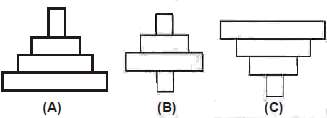Select the correct statement about diagrams A,B,C

Solution:
QUESTION: 140

In an area more than 125000 species of invertebrates,40000 species of plants, 3000 species of fishes, 1300 of birds, 427 of mammals, 427 of amphibians, 378 of reptiles have been reported. Which of the following is wrong about it.

Solution:
QUESTION: 141

Fermentation is differ from aerobic respiration

Solution:
QUESTION: 142

During TCA which of the following intermediate is a result of two sucessive decarboxylations?

Solution:
QUESTION: 143

Minamata and itai-itai are caused by the pollution of :

Solution:
QUESTION: 144

Match the following
(A) Carbohydrate    (I) 1.3
(B) Tripalmitin         (II) 0.9
(C) Proteins            (III) 0.7
(D) Organic acids   (IV) 1.0

Solution:
QUESTION: 145

Potato growing in cold climate or hilly areas are bigger in size this is due to

Solution:
QUESTION: 146

Which of the following diagramrepresentATPsynthesis inmitochondria by chemiosmotic hypothesis
IM= Inner membrane
M=Matrix
OC = Outer Chamber

Solution:
QUESTION: 147

Four respiratory enzymes are given below. Arrange them in increasing order of the carbon number of the substrates on which they act.
(I) Enolase       (II) Aconitase
(III) Fumerase  (IV)Alcohol dehydrogenase

Solution:
QUESTION: 148

The following is a simplified scheme showing the fate of glucose during aerobic and anaerobic respiration. Identify the end products that are formed at stages indicated as A, B, C and D. Identify the correct option from those given below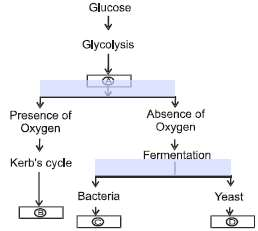Solution:
QUESTION: 149

A molecule of ATP is formed when electron passes from

Solution:
QUESTION: 150

Which of the following is not a crucial event in aerobic respiration

Solution:
QUESTION: 151

Which of the following is most dangerous to wild life?

Solution:
QUESTION: 152

Biomagnification of DDT causes decline in bird population by

Solution:
QUESTION: 153

Indian forest policy (1988) of india has recommonded ....a.....cover for the plains &....b.... for the hills

Solution:
QUESTION: 154

This is metabolic pathway occuring in our cells Label. Substrates from A to G. with the help of following terms.
1. Glycerol  2. Glycolysis 3. Amino Acids 4. Krebs Cycle 5. AcetylCo-A  6. Pyruvate 7. Fatty Acids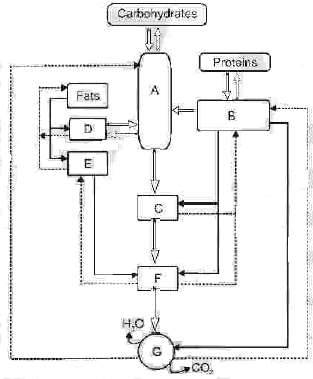Solution:
QUESTION: 155

Respiration is useful for–

Solution:
QUESTION: 156

Water releases protons and 12 molecules of water will release–

Solution:
QUESTION: 157

Injury to plants leads to –

Solution:
QUESTION: 158

How many of the four membrane bound complexes used in mitochondria are proton pumps

Solution:
QUESTION: 159

Number of oxygen atoms required for complete oxidation of one molecule of pyruvic acid is–

Solution:
QUESTION: 160

Aerobic respiration produces 264 gms of CO2 than howmuch CO2 produced fromthe same weight of glucose in alcoholic fermentation

Solution:
QUESTION: 161

The following questions consist of two statements one labelled ASSERTION (a) and the another labelled REASON (R). Select the correct answers to these questions from the codes given below :

Assertion : Tropical rain forest and coral reefs have higher primary productivity.
Reason : They are rich in biodiversity & found in less seasonal environment

Solution:
QUESTION: 162

The following questions consist of two statements one labelled ASSERTION (a) and the another labelled REASON (R). Select the correct answers to these questions from the codes given below :

Assertion : Both cleistogamous flowers and chasmogamous flowers may perform self pollination.
Reason : Only bisexual flowers can proceed it.

Solution:
QUESTION: 163

The following questions consist of two statements one labelled ASSERTION (a) and the another labelled REASON (R). Select the correct answers to these questions from the codes given below :

Assertion: In R-DNA technology the silencing of z-gene of lac operon in E.coli causes blue colour colony of this bacteria in fluorescent dye.
Reason: Colourless colony forms due to insertional inactivation of it.

Solution:
QUESTION: 164

The following questions consist of two statements one labelled ASSERTION (a) and the another labelled REASON (R). Select the correct answers to these questions from the codes given below :

Assertion : Ozone is harmful as well as benefecial.
Reason : It causes global warming, snow blindness as well as provides protection from UV rays.

Solution:
QUESTION: 165

The following questions consist of two statements one labelled ASSERTION (a) and the another labelled REASON (R). Select the correct answers to these questions from the codes given below :

Assertion : Splicing is performed by spliceosomes in hn RNA of eucaryotes.
Reason : Splicing proves the dominance of RNA world.

Solution:
QUESTION: 166

The following questions consist of two statements one labelled ASSERTION (a) and the another labelled REASON (R). Select the correct answers to these questions from the codes given below :

Assertion : Killer strain of Paramoeciumhas kapp particles that remove its sensitive strain inmedium.
Reason : According to gause's principle of competitive exclusion, when two compatible species live together after sometime one of them becomes eliminated

Solution:
QUESTION: 167

The following questions consist of two statements one labelled ASSERTION (a) and the another labelled REASON (R). Select the correct answers to these questions from the codes given below :

Assertion : Agrobacterium tumifaciens is natural genetic engineer in dicot plants only.
Reason : It can be used as biofertilizers for improving production of cereals.

Solution:
QUESTION: 168

The following questions consist of two statements one labelled  ASSERTION (a) and the another labelled REASON (R). Select the correct answers to these questions from the codes given below :

Assertion : In human population, the value of 'r' is higher in a population that shows bell shaped pyramid.
Reason : The number of pre-reproductive & reproductive individuals is approximately same in it.

Solution:
QUESTION: 169

The following questions consist of two statements one labelled ASSERTION (a) and the another labelled REASON (R). Select the correct answers to these questions from the codes given below :

Assertion : Nucellar polyembryony is found in Citrus & Mango.
Reason : In some plants, nucellus remains in seed even after completion of embryonic development

Solution:
QUESTION: 170

The following questions consist of two statements one labelled ASSERTION (a) and the another labelled REASON (R). Select the correct answers to these questions from the codes given below :

Assertion : Pleiotropy and multiple allelism show mendelian inheritance.
Reason : Both regulate inheritance of more than one phenotypes.

Solution:
QUESTION: 171

The following questions consist of two statements one labelled ASSERTION (a) and the another labelled REASON (R). Select the correct answers to these questions from the codes given below :

Assertion : Ubiquinone is one of the important carrier of respiratory ETS.
Reason : Ubiquinone helps in oxidation of both NADH + H+ and FADH2